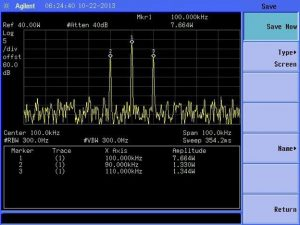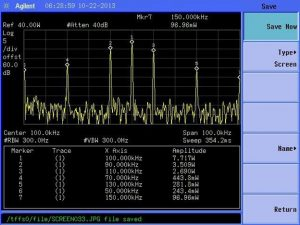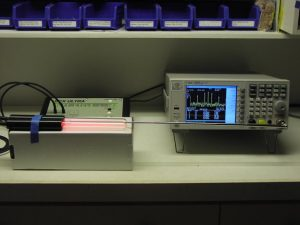# Hand-held Ray Tubes (30 watts) – Test Data Proof

Here we are showing that the BCX Ultra hand-ray tubes OUTPUT 30 WATTS (refer below to the device readouts measuring the power, proving this).

Important to understand how power works. Peak power, Average power, RMS power, Duty Cycle. First, “available” power and “delivered” power are entirely different. The BCX Ultra hand-held raytubes are capable of delivering 30 watts. The more contact there is to them, and the more conductive the material, the more power they deliver. Second, the 30 watts is peak power. All waveforms except square will degrade the power to some extent, and if there is a frequency (which there always is with the rife machine), the average power will be 1/2 because 50% of the time, the hand-held raytubes will be off, and 50% of the time they will be on. So, you can see that with peak power of 30 watts, the average power is never greater than 15 watts, and here is one of the explanations for their misconceptions about our power supply and our output power. In the case of the rife machine, peak power is what counts when delivering a frequency wave type. Why? Because; for example, when at the peak of the square (which is where 100% power delivered as fast as it can for that frequency) is the goal to get a strong/fast surge to perform a kill off of the organisms then it stays at that power at that frequency and then turns off .. In the case of the square wave it turns on 100%  (30 watts) and then turns off power to 0% power (which will be 0  watts) so then taking the average of the ‘on’ power and the ‘off’ power will be 50% of its full power it is capable.

## PROOF

BCX Ultra Raytubes output 30 watts maximum at a carrier frequency of 100KHz. That carrier is modulated by different types of waveforms and frequencies. Modulating the carrier turns it on and off in varying amounts. Thus, the maximum output power of 30 watts is present only part of the time. If you measure the power when the tube is on full, you will see 30 watts. Such is the case when the carrier is modulated with a squarewave at 1 Hz. For 1/2 second, the power is 30 watts, and for the other 1/2 second, there is no power. Below shows the power measurement during the 1/2 second that the tubes are on:When the carrier is modulated at higher frequencies and the measurement time covers both the on and off times, the off time power is averaged in, resulting in lower average power levels. Modulating the carrier creates additional frequencies. Here, the 100KHz carrier is modulated with a sinewave at 10KHz. The resulting reduced power is now distributed between the 100KHz carrier, and two new frequencies at 90KHz and 110KHz:When the modulating waveform is a squarewave, a theoretically infinite number of additional frequencies are produced. The farther away from the carrier that they are, the lower the amount of power each has. Practically, only the first 3 or 4 on either side have any significant power. In the image below, the 100KHz carrier is modulated with a 10KHz squarewave, and the reduced power is shown distributed between the 100KHz carrier, and new frequencies at 50KHz, 70KHz, 90KHz, 110KHz, 130KHz, and 130KHz.